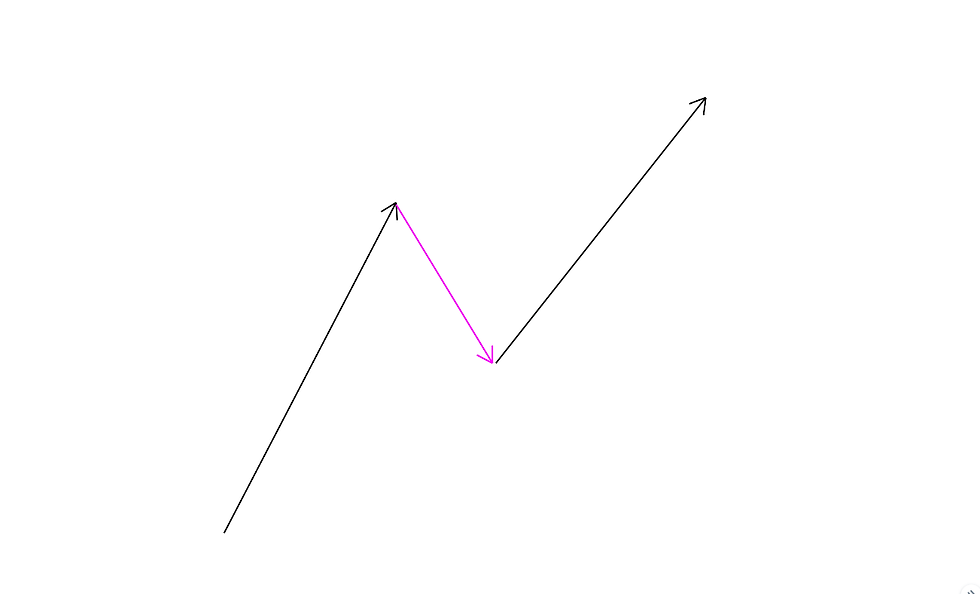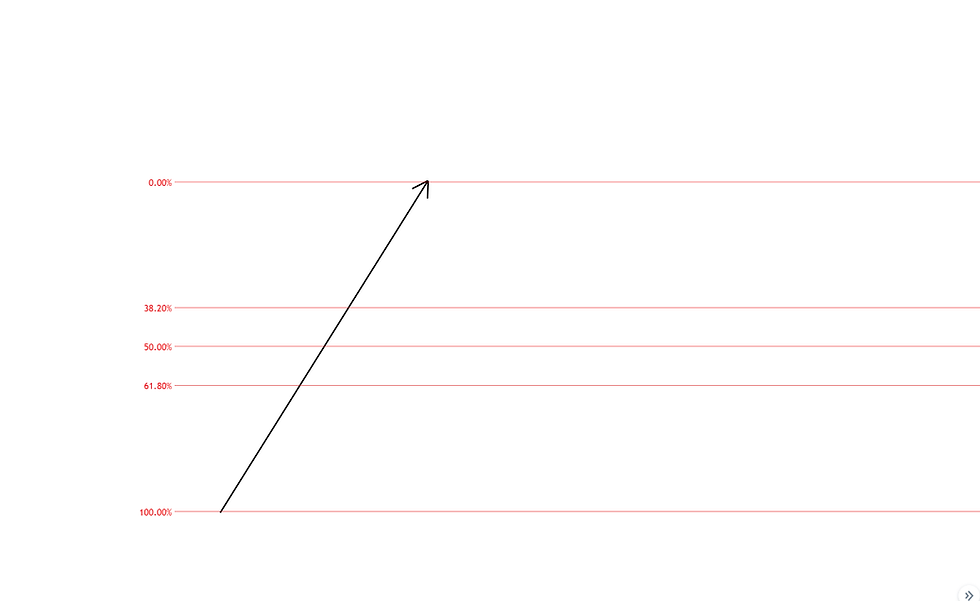top of page
Search

# Fibonacci Retracements and Extentions

The most basic form of Fibonacci analysis concerns retracements and extensions. A retracement is a temporary reversal or correction in a trend. In figure 1, the diagram shows a first leg up which is retraced by the pink arrow, before the prevailing trend continues to move the market higher.Figure 1

The purpose of fibonacci retracement tools is to provide an idea of how far a retracement is likely to go before the prevailing trand regains control. To use the tool, divide a swing into the fibonacci ratios by plotting the tool over the vertical distance from the beginning of the swing to the end, as done in figure 2. The red horizontal lines marking the 38.2%, 50% and 61.8% are all potential price targets where the correction is likely to find support.Figure 2

It may be that the retracement comes down as far as the 38.2% level as shown in the diagram in figure 3. The market could reverse at any of the three levels, or none at all. But Knowing where the retracement levels are gives you and idea of where the market will find some support (or resistance for retracements in bear markets).Figure 3

Figures 4-6 show retracements of 38.2%, 50%, and 61.8% repectively. Each example is taken from the gold market in the last week at the time of writing.Figure 4 - 38.2% retracementFigure 5 - 50% retracementFigure 6 - 61.8% retracement

Fibonacci extensions can be used to generate price targets when a reversal is expected to advance beyond the previous swing. The most common extension levels are 161.8%, 261,8% and 423.6%, however most charting packages will provide more such as 441.4%. The diagram in figure 7 shows how a 161.8% extension may be used as a price target. The red arrow representing the second swing is divided into the Fibonacci ratios. The 161.8% level is below the previous low, and acts as a suitable target for the downtrend.Figure 7

Figure 8 gives a real example of a 161.8% extension, again from the gold market.Figure 8

Fibonacci retracements and extensions should not be considered valid reasons to enter trades in isolation. There are many instances where the market will bulldoze through seemingly every level without hesitation. However, they can be very useful when used in conjunction with other indicators. Remember that simple retracements and extensions are a very small part of Fibonacci Analysis. If mastered in its entirety, Fibonacci Analysis is immensely powerful in identifying the most significant price levels in any market. This is merely a brief introduction which provides little application, but it is a good start.